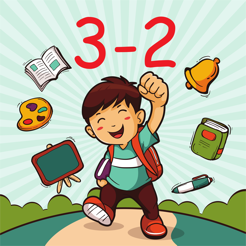## Screenshots

•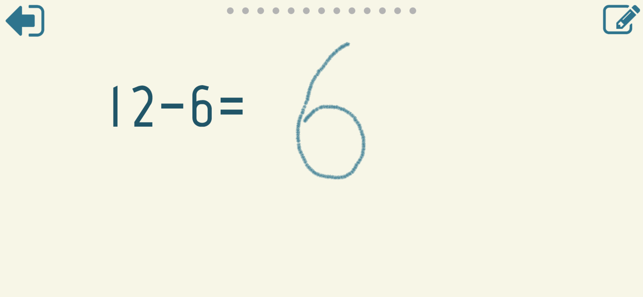•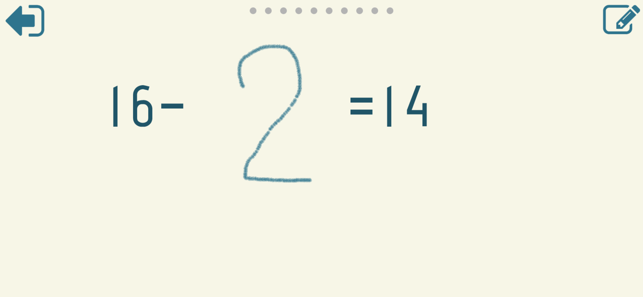•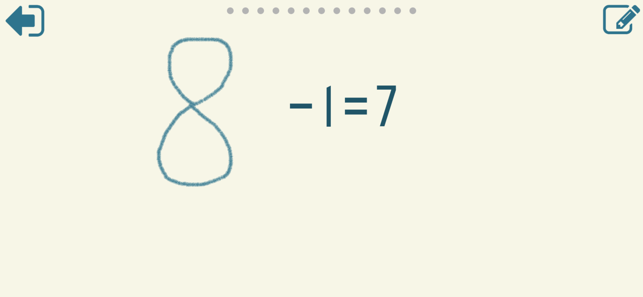•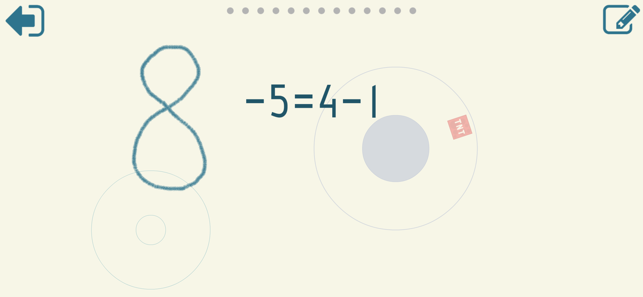•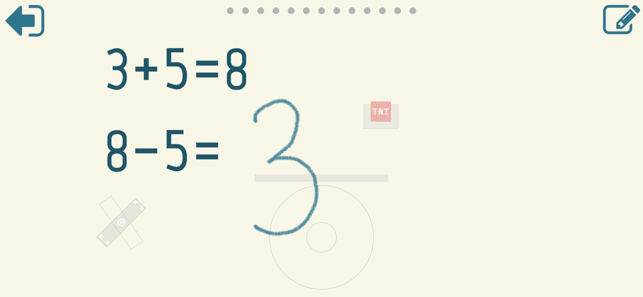•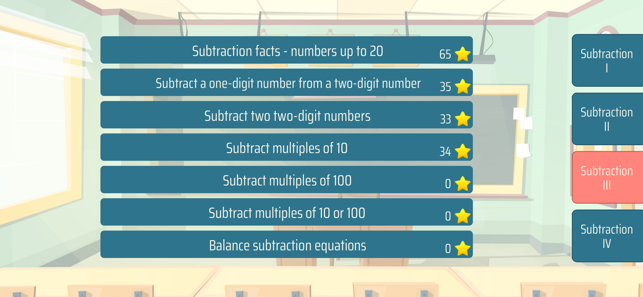•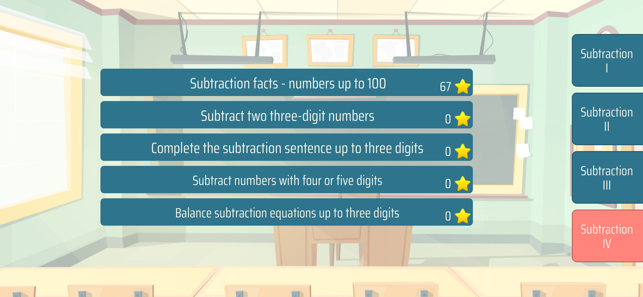•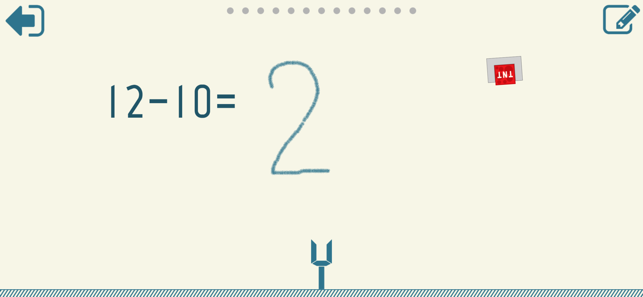•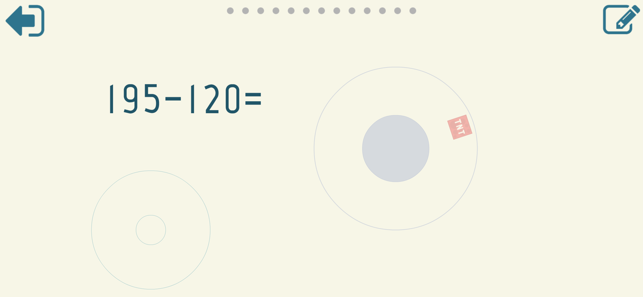•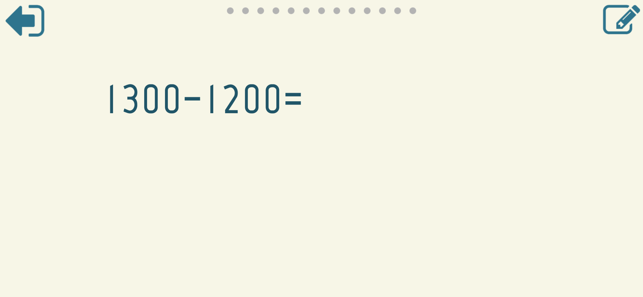## Description

Subtraction Math Trainer is a math learning app designed to help you to practice subtraction. The built-in handwriting recognition allows you to draw answers directly on the screen. Comes with a number of simple mini-games to make learning more effective and less boring.

You can practice the following math skills:

Subtraction facts - numbers up to 10
Subtraction facts - numbers up to 18
Subtract a multiple of ten
Subtraction facts - numbers up to 20
Subtract a one-digit number from a two-digit number
Subtract two two-digit numbers
Subtract multiples of 10
Subtract multiples of 100
Subtract multiples of 10 or 100
Balance subtraction equations
Subtraction facts - numbers up to 100
Subtract two three-digit numbers
Complete the subtraction sentence up to three digits
Subtract numbers with four or five digits
Balance subtraction equations up to three digits

## What’s New

Version 2.0.0

New design. Extended selection of math skills to practice.

## Information

Seller
Sergey Malugin
Size
34 MB
Category
Education
Compatibility

Requires iOS 8.0 or later. Compatible with iPhone, iPad, and iPod touch.

Languages

English, German, Russian

Age Rating
4+
Price
\$1.99

## Supports

•